Function Repository Resource:

# BenchmarkPlot

Plot the timings of a benchmark

Contributed by: Taliesin Beynon
 ResourceFunction["BenchmarkPlot"][f,g] plots the time taken to execute f with input g[n] against integer n, where the n are chosen automatically. ResourceFunction["BenchmarkPlot"][f,g, {n1,n2,…}] uses the explicit ni to generate input g for f. ResourceFunction["BenchmarkPlot"][{f1,f2, …},…] plots the time taken for each of the fi. ResourceFunction["BenchmarkPlot"][data, …] plots the results of a previous ResourceFunction["EvaluateBenchmark"] computation.

## Details and Options

ResourceFunction["BenchmarkPlot"] effectively uses Monitor.

## Examples

### Basic Examples (2)

Get a plot for the inverse of random matrices:

 In:=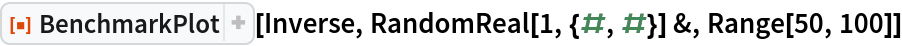Out=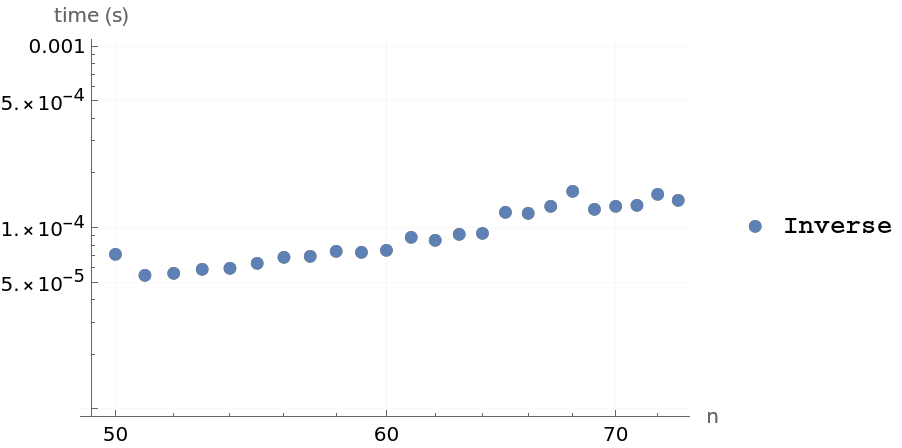Get a plot for the data of a previous benchmark:

 In:=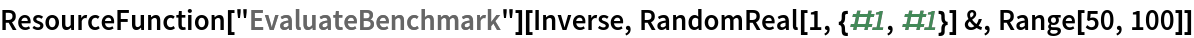Out=In:=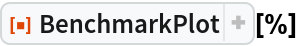Out=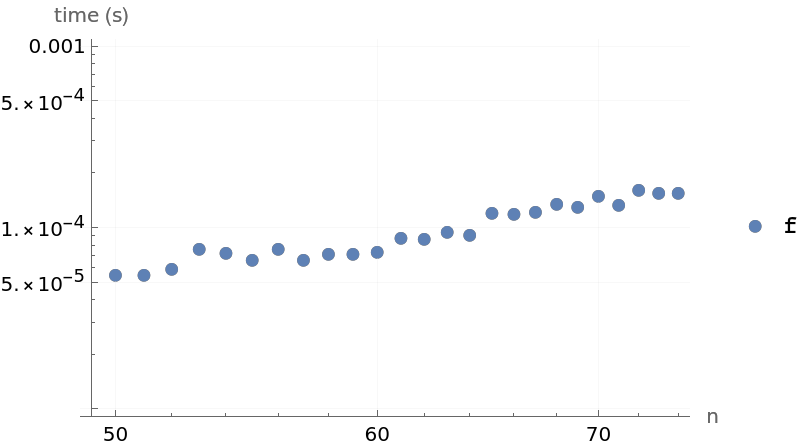### Options (1)

Use "IncludeFits" to get a fit for the data:

 In:=Out=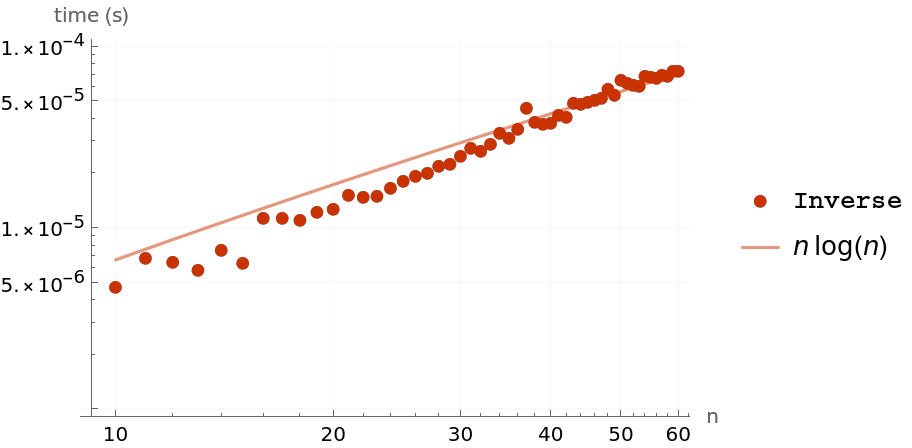## Requirements

Wolfram Language 11.3 (March 2018) or above

## Version History

• 1.0.0 – 06 February 2019